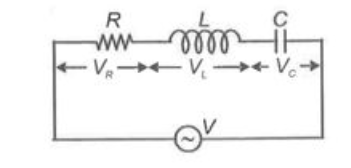Question

Updated on:30/05/2023

# At resonance in series R-L-C circuitText Solution

A
VR+VL+VC=0
B
VR+VL=0
C
VR+VC=0
D
VL+VC=0

Transcript

at resonance in series RLC circuit since this is a series RLC circuit at resonance is series resonance and the resonance of a series RLC circuit occurs when the Inductive and capacitive reactance is are equal in magnitude but cancel Each Other because they are 180 degrees apart in face we can understand this by phasor diagram we know that current in the series circuit is same sote concurrent reference De Sathish voltage across resistance and in resonance real and BC are 180 degrees apart we will we see voltage across inductor and voltage across capacitor NSE CEO b l - VC and the component of resistance
give the voltage supply voltage V so during resonance voltage across inductor is equal to voltage across capacitor tense simple reactances Excel is equal to texy we know that impedance of series RLC circuit is equal to under root a square + x minus b whole square is equal to 110 paath become zero impedance of series RLC circuit is due to the resistance r and the voltage V square is equal to V R square + a minus b whole square
since we're Lage equal to BC during resonance so V square is equal to V R square or supply voltage V is equal to voltage across resistance VR so here we can write wheel + VC will be equal to zero always in resonance and options option A V A + B + BC is equal to zero since real NBC has a magnitude and in opposite direction so they will cancel out Each Other while + BC will be zero but this part is not zero so this is wrong option B B A + B L this part cannot be zero it is also home VR plus we see this part is also wrong because it cannot
option 3 wheel + BC is equal to zero yes this option is correct because we're + BC has a magnitude and in opposite direction so real plus minus VC will be zero option is the correct option